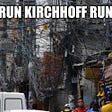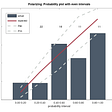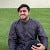# Introduction

When we are faced with a combinatorial optimization problem, our goal is to design an algorithm that satisfies three important properties.

(1) It is efficient, i.e., runs in time polynomial with respect to the input size.

(2) It returns an optimal solution.

(3) It works for every instance of the problem.

However, when we deal with an NP-complete problem, it is not at all clear whether such algorithms that satisfy all three aforementioned properties even exist. So, one natural approach to slightly simplify our lives is to relax one of the three properties. In the case of approximation algorithms, we decide to relax one the properties . This means that we are interested in efficient algorithms that always return feasible solutions, and what we care to bound is the gap between the value of the solution they return and the value of an optimal solution.

# NP-complete

In the computational theory, a problem is called NP-complete when:

• It is a problem in which the solution’s correctness can be proved quickly and the brute-force search algorithm can find a solution to the problem using all the possible solutions.
• The problem can be used to simulate other problems whose solutions’ correctness can be verified quickly.

NP-complete problems have unknown status and no polynomial-time algorithm exists for such problems. However, if one NP-complete problem can be solved within polynomial time, the solution to all other problems can be obtained.

# Definition

An algorithm A is an approximation for a problem L : if given any valid instance I, it finds a solution A(I) for L and A(I) is “close” to optimal solution OPT(I).

Approximation ration (or bound) β of approximation algorithm A for problem L:

A(I)/OPT(I) ≥ β; if L is a minimization problem and A(I) ≥ OPT(I) > 0

OPT(I)/A(I) ≥ β; if L is a maximization problem and OPT(I) ≥ A(I) > 0

# Performance of approximation algorithms

To understand the performance of approximation algorithms, consider the following scenarios:

Scenario 1:

Think of an optimization problem in which every solution has a cost. The aim is to identify the near-optimal solution. Based on the situation, this optimal solution may be the solution with the maximum possible cost or the solution with the least possible cost. This means that the problem will either be a minimization problem or a maximization problem.

Now, let C be the cost of the solution generated for a problem of input size n, and C* be the problem’s optimal solution. Consequently, the approximation ratio P(n) will be given as,

max (C/C*, C*/C) ≤ P(n)

Scenario 2:

If the algorithm reaches an approximation ratio P(n), the algorithm is called the P(n) approximation algorithm.

• For a maximization problem, 0< C < C*, and the ratio of C*/C gives the factor by which the cost of an optimal solution is larger than the cost of the approximate algorithm.
• For a minimization problem, 0< C* < C, and the ratio of C/C* gives the factor by which the cost of an approximate solution is larger than the cost of an optimal solution.

# Examples of approximation algorithm

The examples of an approximation algorithm include:

• The vertex-cover problem
• Traveling salesman problem (TSP)
• The set covering problem

# Vertex cover problem

A vertex cover of a graph is the set of vertices having a minimum of one endpoint for every edge in the graph. In this problem, the optimization problem is about selecting the least number of vertices that cover all the edges in the graph.

The vertex cover problem is NP-Complete because the polynomial-time solution for identifying the minimum vertex cover is unknown. However, there exist approximate polynomial-time algorithms for solving the vertex cover problem.

Below is the approximate algorithm for the vertex cover problem:

• Declare the vertex cover set as empty.
• Name the set of all the edges of graph E.
• While E is not empty, do the following:
• Select an arbitrary edge (u, v) from the set, add its constituent nodes, u and v, to the vertex cover set.
• Remove all the edges having u or v as a part of them from the set E.
• Return the final answer (the vertex cover set) once the set E gets empty.

# Traveling-salesman problem

The traveling-salesman problem is used for finding the shortest route between a set of cities or the locations that the salesman has to visit. The optimization problem in the traveling salesman problem is the one where the salesman has to choose the path that has the least cost so that the cost of traveling and distance traveled are the lowest. The traveling salesman problem is NP-complete as no polynomial-time solution is known for the problem.

Here is the approximate approach used for solving the traveling salesman problem:

• Select any city, say city A, as the start and endpoint for the salesman. This means that the route will be cyclic.
• Now, create all possible permutations of the cities (n-1)!
• Determine the cost of every permutation.
• Track the minimum cost permutation.
• Return the permutation having the least value.

# The set covering problem

For a given universal set U of n elements and a collection of subsets S, the set cover problem aims to find the minimum cost subcollection of S, which consists of all elements of the set U. The set covering problem is one of Karp’s 21 NP-complete problems.

The set cover problem is NP-complete since no polynomial time solution for this problem has been determined. However, a greedy approximate algorithm for the set cover problem exists, which is as mentioned below:

Consider:

U = universal set of elements

{S1, S2, S3, …, Sm} = collection of subsets of U

Cost(S1), Cost(S2), …, Cost(Sm) = cost of subsets

Now, the algorithm will be:

Initialize the set of elements as V.

Until V is not equal to U, do the following:

• Search for the smallest set Sv in {S1, S2, S3, …, Sm} having the least cost-effectiveness. It means the ratio of Cost(Sv) and the number of the newly added element is the lowest.
• Add the elements selected from the above Sv to V. It means performing a union operation between V and Sv.

# Conclusion

The field of approximation algorithms is vast and quite technical, and we only got a glimpse of the arguments that are used to design good approximation algorithms. In my personal opinion, this is an area full of beautiful ideas and techniques.

Authors — Samarth Gawande, Harsh Satdeve, Adnan Shaikh, Kirtish Surangalikar

--

--

--

Love podcasts or audiobooks? Learn on the go with our new app.

## Free Spins Keep What You Win No Deposit 2019## Sieve of Eratosthenes, finding prime numbers upto n## An Introduction to Grover’s Algorithm## An Intuitive Explanation of the Monty Hall Problem## Simply, Kirchhoff’s Laws## Math in Unity - Tracking the mouse cursor## Probability audit## Probability for Pragmatists